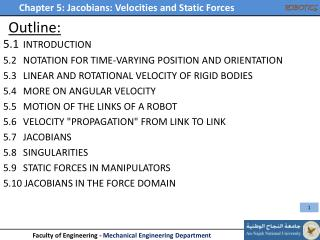DownloadDownload PresentationOutline:

# Outline:

Download Presentation## Outline:

- - - - - - - - - - - - - - - - - - - - - - - - - - - E N D - - - - - - - - - - - - - - - - - - - - - - - - - - -
##### Presentation Transcript

1. Outline: 5.1 INTRODUCTION 5.2 NOTATION FOR TIME-VARYING POSITION AND ORIENTATION 5.3 LINEAR AND ROTATIONAL VELOCITY OF RIGID BODIES 5.4 MORE ON ANGULAR VELOCITY 5.5 MOTION OF THE LINKS OF A ROBOT 5.6 VELOCITY "PROPAGATION" FROM LINK TO LINK 5.7 JACOBIANS 5.8 SINGULARITIES 5.9 STATIC FORCES IN MANIPULATORS 5.10 JACOBIANS IN THE FORCE DOMAIN

2. Introduction • In this Chapter the following concepts will be studied: • The linear and angular velocity of a rigid body to analyze the motion of the manipulator • Forces that act on a rigid body (application on static forces of a manipulator)  Jacobian matrix relations

3. Introduction • Velocity analysis between Cartesian Space and Joint Space Jacobian Matrix Transformation/Mapping • If J is singular • it is not invertible • Singular point/configuration • (Important issue in robot design • that should be avoided) When θ2 = 0/180  singular configuration The Jacobian is important to determine the torque in the joints needed to be applied to give a specific contact force at the End-Effector (Static problem)

4. Notations • Differentiation of a position vector • ≡ the velocity of a position vector • ≡ Linear velocity of a point in space represented by the position vector. Derivative is made relative to frame {B} (Frame of Differentiation) Also the velocity vector is expressed in the same frame {B} To express the velocity in any other frame {A} ≡ The velocity of point (position vector Q) relative the frame {B} expressed in frame {A}. (draw frames for explinations)

5. Notations • Differentiation of a position vector • Often used the velocity of the origin of a frame {C} (for example) relative to the universe frame {U}. Remember that in the differentiation is made relative to {U} and expressed in {U} • as v is small-letter the differentiation is made relative/ respect to {U}, but the velocity is expressed in {A}. • Example:

6. Notations • Example 5.1: